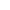Go to content
This display is based on a typical examination question. You can change some of the details in the question and then see the answer, step by step.## Summary/BackgroundYou must be clear about how to find the median, upper quartile and lower quartile from a cumulative frequency graph.

Remember that the Interquartile range = Upper quartile - Lower quartile.You should know how to draw a number of other statistical diagrams too, including:

scatter diagrams, pie charts, bar charts, line graphs, stem & leaf diagrams and box & whisker plotsYou can get a better display of the maths by downloading special TeX fonts from jsMath. In the meantime, we will do the best we can with the fonts you have, but it may not be pretty and some equations may not be rendered correctly.

## Glossary

### frequency

Of a function: the rate of repetition of a periodic function.
In statistics: the number of occurances of a value

### graph

A diagram showing a relationship between two variables.
The diagram shows a vertical y axis and a horizontal x axis.

### interquartile range

A measure of dispersion; the difference between the upper and lower quartiles; Q3-Q1.

### median

the middle value of a set of data that has been arranged in order of magnitude; half the data values are above it and half are below.

### quartile

In an ordered set of data 25% are below the first (Q1), 50% are below the second (Q2), 75% are below the third (Q3).

### range

In Statistics: the difference between the largest and smallest values in a data set; a simple measure of spread or variation
In Pure Maths: the values that y can take given an equation y=f(x) and a domain for x.

### union

The union of two sets A and B is the set containing all the elements of A and B.

Full Glossary List

## This question appears in the following syllabi:

SyllabusModuleSectionTopicExam Year
AQA AS Maths 2017StatisticsData Presentation and InterpretationCumulative Frequency-
AQA AS/A2 Maths 2017StatisticsData Presentation and InterpretationCumulative Frequency-
AQA GCSE (9-1) Higher (UK)S: StatisticsS3: Grouped Data, Histograms and Cumulative FrequencyCumulative Frequency-
CBSE X (India)Statistics and ProbabilityStatisticsCumulative frequency graph-
CIE IGCSE (9-1) Maths (0626 UK)9 StatisticsE9.7 Cumulative Frequency and Box-PlotsCumulative Frequency-
Edexcel AS Maths 2017StatisticsData Presentation and InterpretationCumulative Frequency-
Edexcel AS/A2 Maths 2017StatisticsData Presentation and InterpretationCumulative Frequency-
Edexcel GCSE (9-1) Higher (UK)S: StatisticsS3: Grouped Data, Histograms and Cumulative FrequencyCumulative Frequency-
GCSE Higher (UK)StatisticsDisplaying dataCumulative frequency-
OCR AS Maths 2017StatisticsWorking with DataCumulative Frequency-
OCR GCSE (9-1) Higher (UK)12: Statistics12.02b: Grouped DataCumulative Frequency-
OCR MEI AS Maths 2017StatisticsWorking with DataCumulative Frequency-
Universal (all site questions)DDisplaying dataCumulative frequency-